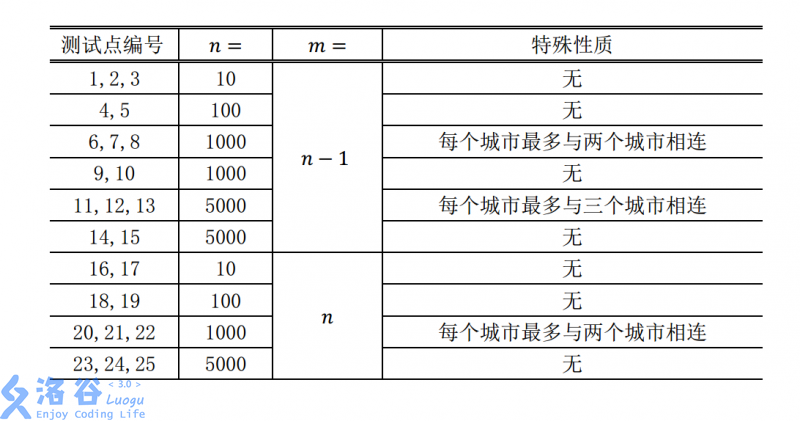# P5022 旅行

• 3K通过
• 24K提交
• 题目提供者 龟龟号打捞船 铲屎官
• 评测方式 云端评测
• 标签 搜索 深度优先搜索,DFS 贪心 NOIp提高组 2018
• 难度 提高+/省选-
• 时空限制 1000ms / 128MB
• 提示：收藏到任务计划后，可在首页查看。

## 题目描述

小 Y 是一个爱好旅行的 OIer。她来到 X 国，打算将各个城市都玩一遍。

小Y了解到， X国的 $n$ 个城市之间有 $m$ 条双向道路。每条双向道路连接两个城市。 不存在两条连接同一对城市的道路，也不存在一条连接一个城市和它本身的道路。并且， 从任意一个城市出发，通过这些道路都可以到达任意一个其他城市。小 Y 只能通过这些 道路从一个城市前往另一个城市。

小 Y 的旅行方案是这样的：任意选定一个城市作为起点，然后从起点开始，每次可 以选择一条与当前城市相连的道路，走向一个没有去过的城市，或者沿着第一次访问该 城市时经过的道路后退到上一个城市。当小 Y 回到起点时，她可以选择结束这次旅行或 继续旅行。需要注意的是，小 Y 要求在旅行方案中，每个城市都被访问到。

为了让自己的旅行更有意义，小 Y 决定在每到达一个新的城市（包括起点）时，将 它的编号记录下来。她知道这样会形成一个长度为 $n$ 的序列。她希望这个序列的字典序 最小，你能帮帮她吗？ 对于两个长度均为 $n$ 的序列 $A$ 和 $B$，当且仅当存在一个正整数 $x$，满足以下条件时， 我们说序列 $A$ 的字典序小于 $B$。

• 对于任意正整数 $1 ≤ i < x$，序列 $A$ 的第 $i$ 个元素 $A_i$ 和序列 $B$ 的第 $i$ 个元素 $B_i$ 相同。
• 序列 $A$ 的第 $x$ 个元素的值小于序列 $B$ 的第 $x$ 个元素的值。

## 输入输出格式

输入格式：

输入文件共 $m + 1$ 行。第一行包含两个整数 $n,m(m ≤ n)$，中间用一个空格分隔。

接下来 m 行，每行包含两个整数 $u,v (1 ≤ u,v ≤ n)$ ，表示编号为 $u$ 和 $v$ 的城市之 间有一条道路，两个整数之间用一个空格分隔。

输出格式：

输出文件包含一行，$n$ 个整数，表示字典序最小的序列。相邻两个整数之间用一个 空格分隔。

## 输入输出样例

输入样例#1： 复制
6 5
1 3
2 3
2 5
3 4
4 6
输出样例#1： 复制
1 3 2 5 4 6
输入样例#2： 复制
6 6
1 3
2 3
2 5
3 4
4 5
4 6
输出样例#2： 复制
1 3 2 4 5 6

## 说明

【数据规模与约定】

对于 $100\%$ 的数据和所有样例， $1 \le n \le 5000$ 且 $m = n − 1$ 或 $m = n$ 。

对于不同的测试点， 我们约定数据的规模如下：提示
标程仅供做题后或实在无思路时参考。
请自觉、自律地使用该功能并请对自己的学习负责。
如果发现恶意抄袭标程，将按照I类违反进行处理。